Updating search results...

# 71 Results

View
Selected filters:
• Mathematics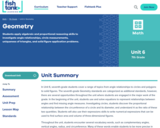Conditional Remix & Share Permitted
CC BY-NC-SA
Rating
0.0 stars

Students apply algebraic and proportional reasoning skills to investigate angle relationships, circle measurements, uniqueness of triangles, and solid figure application problems.

Subject:
Mathematics
Material Type:
Unit of Study
Provider:
Fishtank Learning
Provider Set:
Mathematics
11/19/2021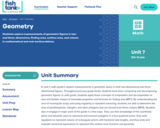Conditional Remix & Share Permitted
CC BY-NC-SA
Rating
0.0 stars

Students explore measurements of geometric figures in two-and three-dimensions, finding area, surface area, and volume in mathematical and real-world problems.

Subject:
Mathematics
Material Type:
Unit of Study
Provider:
Fishtank Learning
Provider Set:
Mathematics
11/19/2021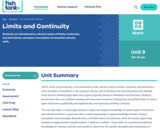Conditional Remix & Share Permitted
CC BY-NC-SA
Rating
0.0 stars

Students are introduced to calculus topics of limits, continuity, and derivatives, and gain a foundation of essential calculus skills.

Subject:
Algebra
Mathematics
Material Type:
Unit of Study
Provider:
Fishtank Learning
Provider Set:
Mathematics
11/19/2021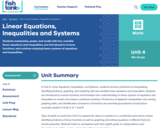Conditional Remix & Share Permitted
CC BY-NC-SA
Rating
0.0 stars

Students manipulate, graph, and model with two-variable linear equations and inequalities, are introduced to inverse functions, and continue studying linear systems of equations and inequalities.

Subject:
Algebra
Mathematics
Material Type:
Unit of Study
Provider:
Fishtank Learning
Provider Set:
Mathematics
11/19/2021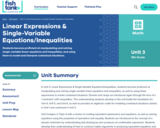Conditional Remix & Share Permitted
CC BY-NC-SA
Rating
0.0 stars

Students become proficient at manipulating and solving single-variable linear equations and inequalities, and using them to model and interpret contextual situations.

Subject:
Algebra
Mathematics
Material Type:
Unit of Study
Provider:
Fishtank Learning
Provider Set:
Mathematics
11/19/2021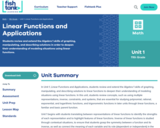Conditional Remix & Share Permitted
CC BY-NC-SA
Rating
0.0 stars

Students review and extend the Algebra 1 skills of graphing, manipulating, and describing solutions in order to deepen their understanding of modeling situations using linear functions.

Subject:
Algebra
Mathematics
Material Type:
Unit of Study
Provider:
Fishtank Learning
Provider Set:
Mathematics
11/19/2021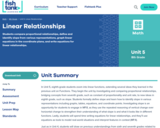Conditional Remix & Share Permitted
CC BY-NC-SA
Rating
0.0 stars

Students compare proportional relationships, define and identify slope from various representations, graph linear equations in the coordinate plane, and write equations for linear relationships.

Subject:
Mathematics
Material Type:
Unit of Study
Provider:
Fishtank Learning
Provider Set:
Mathematics
11/19/2021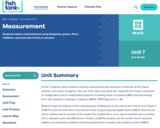Conditional Remix & Share Permitted
CC BY-NC-SA
Rating
0.0 stars

Students explore measurement using kilograms, grams, liters, milliliters, and intervals of time in minutes.

Subject:
Mathematics
Material Type:
Unit of Study
Provider:
Fishtank Learning
Provider Set:
Mathematics
11/19/2021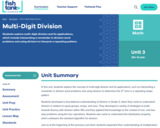Conditional Remix & Share Permitted
CC BY-NC-SA
Rating
0.0 stars

Students explore multi-digit division and its applications, which include interpreting a remainder in division word problems and using division to interpret a repeating pattern.

Subject:
Mathematics
Material Type:
Unit of Study
Provider:
Fishtank Learning
Provider Set:
Mathematics
11/19/2021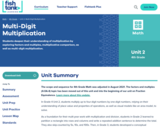Conditional Remix & Share Permitted
CC BY-NC-SA
Rating
0.0 stars

Students deepen their understanding of multiplication by exploring factors and multiples, multiplicative comparison, as well as multi-digit multiplication.

Subject:
Mathematics
Material Type:
Unit of Study
Provider:
Fishtank Learning
Provider Set:
Mathematics
11/19/2021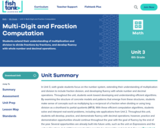Conditional Remix & Share Permitted
CC BY-NC-SA
Rating
0.0 stars

Students extend their understanding of multiplication and division to divide fractions by fractions, and develop fluency with whole number and decimal operations.

Subject:
Mathematics
Material Type:
Unit of Study
Provider:
Fishtank Learning
Provider Set:
Mathematics
11/19/2021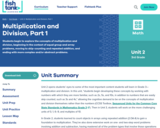Conditional Remix & Share Permitted
CC BY-NC-SA
Rating
0.0 stars

Students begin to explore the concepts of multiplication and division, beginning in the context of equal group and array problems, moving to skip-counting and repeated addition, and ending with more complex and/or abstract problems.

Subject:
Mathematics
Material Type:
Unit of Study
Provider:
Fishtank Learning
Provider Set:
Mathematics
11/19/2021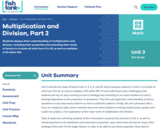Conditional Remix & Share Permitted
CC BY-NC-SA
Rating
0.0 stars

Students deepen their understanding of multiplication and division, including their properties and extending their study of factors to include all units from 0 to 10, as well as multiples of 10 within 100.

Subject:
Mathematics
Material Type:
Unit of Study
Provider:
Fishtank Learning
Provider Set:
Mathematics
11/19/2021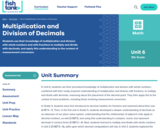Conditional Remix & Share Permitted
CC BY-NC-SA
Rating
0.0 stars

Students use their knowledge of multiplication and division with whole numbers and with fractions to multiply and divide with decimals, and apply this understanding to the context of measurement conversion.

Subject:
Mathematics
Material Type:
Unit of Study
Provider:
Fishtank Learning
Provider Set:
Mathematics
11/19/2021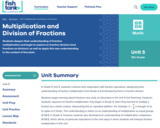Conditional Remix & Share Permitted
CC BY-NC-SA
Rating
0.0 stars

Students deepen their understanding of fraction multiplication and begin to explore to fraction division (and fractions as division), as well as apply this new understanding to the context of line plots.

Subject:
Mathematics
Material Type:
Unit of Study
Provider:
Fishtank Learning
Provider Set:
Mathematics
11/19/2021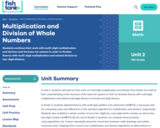Conditional Remix & Share Permitted
CC BY-NC-SA
Rating
0.0 stars

Students continue their work with multi-digit multiplication and division and the base-ten system in order to finalize fluency with multi-digit multiplication and extend division to two-digit divisors.

Subject:
Mathematics
Material Type:
Unit of Study
Provider:
Fishtank Learning
Provider Set:
Mathematics
11/19/2021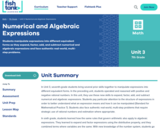Conditional Remix & Share Permitted
CC BY-NC-SA
Rating
0.0 stars

Students manipulate expressions into different equivalent forms as they expand, factor, add, and subtract numerical and algebraic expressions and face authentic real-world, multi-step problems.

Subject:
Mathematics
Material Type:
Unit of Study
Provider:
Fishtank Learning
Provider Set:
Mathematics
11/19/2021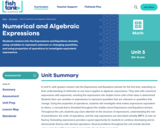Conditional Remix & Share Permitted
CC BY-NC-SA
Rating
0.0 stars

Students venture into the Expressions and Equations domain, using variables to represent unknown or changing quantities, and using properties of operations to investigate equivalent expressions.

Subject:
Mathematics
Material Type:
Unit of Study
Provider:
Fishtank Learning
Provider Set:
Mathematics
11/19/2021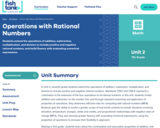Conditional Remix & Share Permitted
CC BY-NC-SA
Rating
0.0 stars

Students extend the operations of addition, subtraction, multiplication, and division to include positive and negative rational numbers, and build fluency with evaluating numerical expressions.

Subject:
Mathematics
Material Type:
Unit of Study
Provider:
Fishtank Learning
Provider Set:
Mathematics
11/19/2021Conditional Remix & Share Permitted
CC BY-NC-SA
Rating
0.0 stars

Students are introduced to the coordinate plane and use it to represent the location of objects in space, as well as to represent patterns and real-world situations.

Subject:
Mathematics
Material Type:
Unit of Study
Provider:
Fishtank Learning
Provider Set:
Mathematics